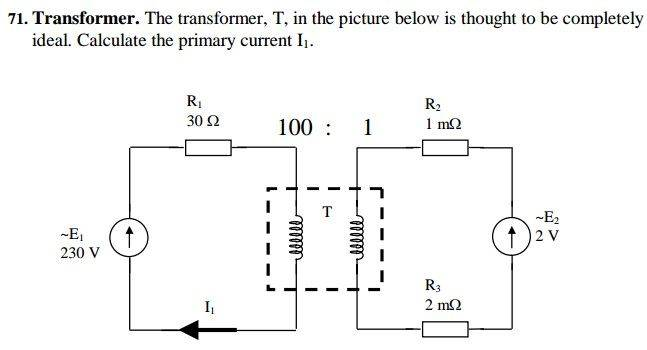# Quick question about transformer circuit

• loki4000
In summary, the conversation is about the correctness of a solution for a problem involving equations and current calculations. The initial solution was found to be incorrect and a new solution was presented and checked. The final answer for the primary current was determined to be 0.5 A.

## Homework Statement

Can someone inform me wheher my solution is correct?## Homework Equations

V1 = E2 * (10^-3)/2*(10^-3) = 0.001
V2 = E1
Ra = (R2+R3) = 0.02
Rb = R1 = 30
n = (1/100)
n^2 = (1/100)^2

## The Attempt at a Solution

I1 = (V'1 - V2)/(Ra'+Rb)
I1 = (0.001/n - 230)/(0.02/n^2 + 30)
I1=-0.9995652174

loki4000 said:
Ra = (R2+R3) = 0.02
Why is this sum equated to 20mΩ ?

NascentOxygen said:
Why is this sum equated to 20mΩ ?
Yup, my error.
Anyway, after looking at problem again I solved it in different way. I think this way makes more sense (at least to me).
Previous solution did not count ).
So, can you tell me whether this one correct?

I2 = current over R2
a=100/1=100
I2=aI1
V2=V1/a
V2 = (-I1R1+E)/a
V2 = (-I2R2)+(E2* (R2/(R1+R2)))
(-I2R2)+(E2* (R2/(R1+R2)))=(-I1R1+E)/a

-(10^-3)I2+(0.6667)=-(3/10)I1+2.30

-0.1I1+(0.6667)=-(3/100)I1+2.30
-0.1I1+(3/10)I1=+2.30-(0.6667)
0.2I1 = 1.6333
I1 = 8.1665

loki4000, you really don't need anyone else to check your answer---because you can do the check yourself. Substitute your answer and see what the figures look like.

For example, you determined the primary current to be a little over 8A? What voltage loss would that give you across the primary's resistance, and does that sound reasonable?

I think you should first explain in words how you will go about solving this. It would help you, and it will be easier for others to follow what you are doing.

Never mind. I solved it. Thanx for reply anyway.

loki4000 said:
Never mind. I solved it. Thanx for reply anyway.
What answer did you get for the primary current?

I1=0.5 A

loki4000 said:
I1=0.5 A
That is my answer, too.